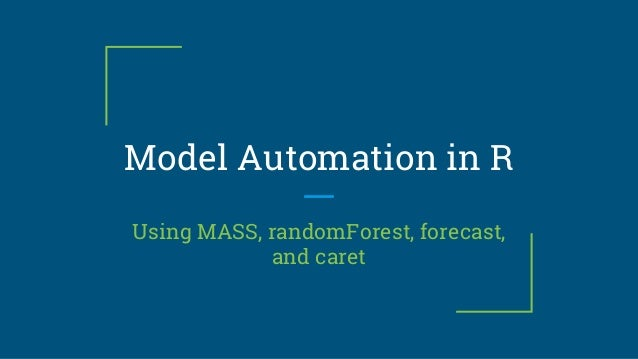Successfully reported this slideshow.Upcoming SlideShare
×

# Model Automation in R

Develop predictive models in R using packages like MASS, caret, randomForest, and forecast from regression to time series.

• Full Name
Comment goes here.

Are you sure you want to Yes No• Be the first to like this

### Model Automation in R

1. 1. Model Automation in R Using MASS, randomForest, forecast, and caret
2. 2. Who is Will Johnson? ● Database Manager at Uline (Pleasant Prairie) ● MS Predictive Analytics (2015) ● Operating www.LearnByMarketing.com ○ R tutorials, thoughts on analysis. Learn By Marketing.com
3. 3. Agenda 1. What is Model Automation 2. Pros and Cons of Model Automation 3. Decision Trees and Random Forests {randomForest} 4. Stepwise Regression {MASS} 5. Auto.Arima for time series {forecast} 6. Hyperparameter Search {caret}
4. 4. What is Model Automation? Hypothesis Space vs Hyperparameter Space
5. 5. Pros and Cons of Model Automation PROS: ● You Don’t Have to Think! ● “Faster” Iterations. ● See what’s “Important” CONS: ● You Don’t Have to Think! ● Jellybeans
6. 6. Agenda 1. What is Model Automation 2. Pros and Cons of Model Automation 3. Decision Trees and Random Forests {randomForest} 4. Stepwise Regression {MASS} 5. Auto.Arima for time series {forecast} 6. Hyperparameter Search {caret}
7. 7. Decision Trees ● Gini Index + Entropy
8. 8. randomForest ● Mean Decrease in Gini Index library(randomForest) rf <- randomForest(y~., data = dat) rf\$importance #Var Name + Importance varImpPlot(rf) #Visualization
9. 9. Stepwise Regression ● AIC
10. 10. Stepwise Regression library(MASS) mod <- lm(hp~.,data=mt) #Step Backward and remove one variable at a time stepAIC(mod,direction = "backward",trace = T) #Create a model using only the intercept mod_lower = lm(hp~1,data=mt) #Step Forward and add one variable at a time stepAIC(mod_lower,direction = "forward", scope=list(upper=upper_form,lower=~1)) #Step Forward or Backward each step starting with a intercept model stepAIC(mod_lower,direction = "both", scope=list(upper=upper_form,lower=~1)) #Get the Independent Variables #(and exclude hp dependent variable) indep_vars <-paste(names(mt)[-which(names(mt)=="hp")], collapse="+") #Turn those variable names into a formula upper_form = formula(paste("~",indep_vars,collapse="")) #~mpg + cyl + disp + drat + wt + qsec + vs + am + gear + carb
11. 11. Auto.Arima ● Time Series models. ● AutoRegressive… ● Moving Averages… ● With Differencing! library(forecast) library(fpp) #Step Backward and remove one variable at a time data("elecequip") ee <- elecequip[1:180] model <- auto.arima(ee,stationary = T) # ar1 ma1 ma2 ma3 intercept #0.8428 -0.6571 -0.1753 0.6353 95.7265 #s.e. 0.0431 0.0537 0.0573 0.0561 3.2223 plot(forecast(model,h=10)) lines(x = 181:191, y= elecequip[181:191], type = 'l', col = 'red')
12. 12. Auto.Arima
13. 13. train {caret} library(caret) #Step Backward and remove one variable at a time tctrl <- trainControl(method = "cv",number=10, repeats=10) rpart_opts <- expand.grid(cp = seq(0.0,0.01, by = 0.001)) rpart_model <- train(y~. data, method="rpart", metric = "Kappa", trControl = tctrl, tuneGrid = rpart_opts, subset = train_log)
14. 14. train {caret}
15. 15. Recap Learn By Marketing.com library(randomForest) varImpPlot() library(MASS) stepAIC() library(forecast) auto.arima() library(caret) train()
16. 16. Questions? Learn By Marketing.com